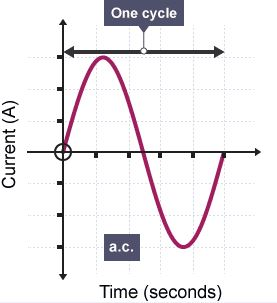## Pages

### Alternating current

Here, we discuss about the alternating current (A.C).

Alternating Current:

"An alternating current is the flow of electric charge that periodically reverses."

Here's an illustration of A.C.

Graphically its as follows,image credit: bbc

The graph above has the same shape as the graphs used to represent simple harmonic motion, and it can be interpreted in the same way. The electrons, in a wire carrying a.c thus move back and forth with SHM. The current varies like a sine wave and so it is described as sinusoidal .

Mathematically,
$$I=I_{0}sin(\omega t)$$
Where,
$\omega$=angular frequency of the supply
$I_{0}$=The peak value of the alternating current (The amplitude of the graph)

And
$$V=V_{0}sin(\omega t)$$

Where:
$V_{0}$ = The peak of the voltage
$\omega$ = angular frequency

Calculating Power in A.C,

Before we define power, it is important that we define Root Mean Square value. $I_{rms}$ or root mean square current is the average value that is equivalent to the value of the steady direct current which dissipates energy in a given resistance at the same rate as the alternating current.

If  $I_{rms}$ value of an a.c. is the current , then the average power $<P>$ dissipated in a load of the resistance $R$ is given by,
$$<P>=I_{rms}^{2}R=\frac{V_{rms}^{2}}{R}$$

Mathematical relationship between the peak value $I_{0}$ of the alternating current and d.c current which delivers the same average electrical power is as follows,

$$I_{rms}=\frac{I_{0}}{\sqrt{2}}$$
Similarly for $V_{rms}$ ,
$$V_{rms}=\frac{V_{0}}{\sqrt{2}}$$

WORKED EXAMPLE

A sinusoidal p.d of peak value $25V$ is connected across $20\Omega$ resistor. What is the average power dissipated in the resistor.

STEP 1: Calculate the r.m.s value os the p.d, $V_{rms}=V_{0}/ \sqrt{2}$
$V_{rms}= 25/√2$
$V_{rms}=17.7V$

STEP 2: Now calculate the average power dissipated using, $P=V^{2}/R$

P=17.72/20
P=15.6W

(Note: if we had used $V_{0}$ instead of Vrms we would’ve got P as $31.3W$, which is double the correct answer)

Note: In collaboration with Kartikay Buchar.